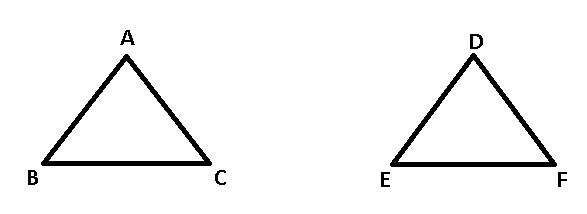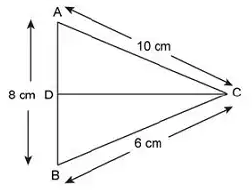0
154

Here you can download CMAT 2022 – important Triangles Questions PDF by Cracku. Very Important Triangles Questions for CMAT 2022 based on asked questions in previous exam papers. These questions will help your CMAT preparation. So kindly download the PDF for reference and do more practice.

Take Free CMAT mock tests here

Question 1: In two similar triangles ABC and MNP, if AB = 2.25 cm, MP = 4.5 cm and PN = 7.5 cm and m ∠ACB = m ∠MNP and m ∠ABC = m ∠MPN, then the length of side BC , in cm, is

a) 4.5

b) 3.75

c) 4.75

d) 3.5

Question 2: The heights of two similar right-angled triangles ΔLMN and ΔOPQ are 48 cm and 36 cm. If OP = 12 cm, then LM is

a) $\frac{10\sqrt{6}}{3}$

b) 16 cm

c) 20 cm

d) 12 cm

Question 3: Taking any three of the line segments out of segments of length 2 cm, 3 cm, 5 cm and 6 cm, the number of triangles that can be formed is :

a) 3

b) 2

c) 1

d) 4

Question 4: Two triangles ABC and PQR are congruent. If the area of A ABC is 60 sq. cm, then area of A PQR will be

a) 60 sq.cm

b) 30 sq.cm

c) 15 sq.cm

d) 120 sq.cm

Question 5: The perimeters of two similar triangles ΔABC andCare 36cm and 24 cm respectively. If PQ = 10 cm, then AB is:

a) 25 cm

b) 10 cm

c) 15 cm

d) 20 cm

Question 6: Δ DEF and Δ GHI are similar triangles. Length of DE is 4 cm and length of the corresponding side GH is 9 cm. What is the ratio of areas of Δ DEF and Δ GHI?

a) 81:16

b) 4:9

c) 16:81

d) 9:4

Question 7: Δ ABC and Δ DEF are similar triangles. Length of AB is 10 cm and length of the corresponding side DE is 6 cm. What is the ratio of Perimeter of ΔABC to ΔDEF?

a) 5:3

b) 3:5

c) 25:9

d) 9:25

Question 8: The diagonals do not form at least two congruent triangles in a …………….

a) Parallelogram

b) Rhombus

c) Trapezium

d) Kite

Question 9: If the areas of two similar triangle are in the ratio 5 : 7, then what is the ratio of the corresponding sides of these two triangles?

a) $5 : 7$

b) $25 : 49$

c) $\sqrt5 : \sqrt7$

d) $125 : 343$

Question 10: ΔPQR has sides PQ and PR measuring 983 and 893 units respectively. How many such triangles are possible with all integral sides?

a) 1876

b) 90

c) 1785

d) 1786

Question 11: The areas of two similar triangles ΔABC and ΔPQR are 121 sq cms and 64 sq cms respectively. If PQ = 12 cm, what is the length (in cm) of AB?

a) 7.25

b) 6.25

c) 16.5

d) 5.25

Question 12: The areas of two similar triangles ΔABC and ΔPQR are 36 sq cms and 9 sq cms respectively. If PQ = 4 cm then what is the length of AB (in cm)?

a) 16

b) 12

c) 8

d) 6

Question 13: The perimeter of two similar triangles ABC and PQR are 36 cms and 24 cms respectively. If PQ = 10 cm then the length of AB is

a) 18 cm

b) 12 cm

c) 15 cm

d) 30 cm

Question 14: ∆ABC and ∆DEF are two similar triangles and the perimeter of ∆ABC and ∆DEF are 30 cm and 18 cm respectively. If length of DE = 36 cm, then length of AB is

a) 60 cm

b) 40 cm

c) 45 cm

d) 50 cm

Question 15: If the triangle  ABC and DEF follows the given equation, then these two triangles are similar by which of the following criterion ?
$\frac{AB}{DE}=\frac{BC}{EF}=\frac{AC}{DF}$

a) SAS similarity

b) SSS similarity

c) AAA similarity

d) None of the these

Question 16: Which of the following is the CORRECT option for the triangles having sides in the ratio of 3:4:6?

a) Acute angled

b) Obtuse angled

c) Right angled

d) Either acute or right angled

Question 17: If the ratio of the angle bisector segments of the two equiangular triangles are in the ratio of 3:2 then what is the ratio of the corresponding sides of the two triangles?

a) 2:3

b) 3:2

c) 6:4

d) 4:6

Question 18: If the $\angle A=\angle D$ and $\frac{AB}{DE}=\frac{AC}{DF}$ then both triangles ABC and DEF is similar by which of the following criteria?

a) SAS similarity

b) ASA similarity

c) AAA similarity

d) None of these

Question 19: In a triangle ABC, a line is drawn from C which bisects AB at point D. Find the ratio of area of the triangles DBC and ABC.

a) 1:1

b) 2:1

c) 1:2

d) 1:3

Question 20: Consider the following two triangles as shown in the figure below

a) $\triangle BAC \sim\triangle NMP$

b) $\triangle BAC \sim\triangle MNP$

c) $\triangle CAB \sim\triangle NMP$

d) $\triangle BAC \sim\triangle PMN$

As we can see, the 2 triangles are similar. Their sides are in the ratio 2:1 (MPN: ABC). Hence, BC will be 7.5/2 = 3.75 cm. Option B is the right answer.

ratio of heights of two similar triangles is always equal to the ratio of the corresponding sides of the triangles

i.e, $\frac{\text{height of } \triangle LMN}{\text{height of } \triangle OPQ}$ = $\frac{\text{length of LM}}{\text{length of OP}}$

put in values from the question

$\frac{48}{36}$ = $\frac{\text{length of LM}}{12}$

length of LM = 16

We have to select three values out of the four length values given so that sum of any two values in the chosen set is larger than the third value.

=> Three out of four combination problem and we can choose in $C_3^4$ = 4 ways.

But because of the triangle formation constraint, sides 2cm, 3cm can’t be taken together in any choice.

This reduces number of combinations by 2, [2, 3, 5] and [2, 3, 6]

=> The only two possibilities, [2, 5, 6] and [3, 5, 6].

Ans – (B)

Two congruent triangles are always equal in area.

$\because$ $\triangle$ABC $\cong$ $\triangle$PQR

=> area($\triangle$ABC) = area($\triangle$PQR) = 60 $cm^2$

In Similar triangles , corresponding sides are of same proportion.
$\frac{ Perimeter ΔABC }{ Perimeter ΔPQR }=\frac{AB}{PR}$
$\frac{ 36 }{ 24 }=\frac{AB}{10}$
Ab = 15

It is given that ΔDEF $\sim$ ΔGHI

Also, length of DE = 4 cm and length of the corresponding side GH = 9 cm

=> Ratio of Area of ΔDEF : Area of ΔGHI = Ratio of square of corresponding sides = $(DE)^2$ : $(GH)^2$

= $\frac{(4)^2}{(9)^2} = \frac{16}{81}$

$\therefore$ The required ratio is 16 : 81

=> Ans – (C)

It is given that ΔAB $\sim$ ΔDEF

Also, length of AB = 10 cm and length of the corresponding side DE = 6 cm

=> Ratio of Perimeter of ΔABC : Perimeter of ΔDEF = Ratio of corresponding sides = AB : DE

= $\frac{10}{6} = \frac{5}{3}$

$\therefore$ The required ratio is 5 : 3

=> Ans – (A)

In a parallelogram, rhombus or kite, both pairs of opposite sides are parallel, and thus there are at least two congruent triangles which is not the case in a trapezium which has only one pair of parallel sides.

=> Ans – (C)

Ratio of areas of two similar triangles is equal to the ratio of square of the corresponding sides.

Let the ratio of corresponding sides = $\frac{x}{y}$

=> $\frac{x^2}{y^2}=\frac{5}{7}$

=> $(\frac{x}{y})^2=\frac{5}{7}$

=> $\frac{x}{y}=\frac{\sqrt5}{\sqrt7}$

=> Ans – (C)

Let us assume that the length of the third side is x units.

Case 1: When the side PQ is the largest among three sides.
893 + x > 983
x > 90

Case 2: When the side QR is the largest among the three sides.
893 + 983 > x
x < 1876
Hence we can say that x $\epsilon$ (90, 1876)

Hence, the number of with integral value =  1875 – 91 +1 = 1785.

It is given that ΔABC $\sim$ ΔPQR

Let length of AB = $x$ cm and length of the corresponding side PQ = 12 cm

=> Ratio of Area of ΔABC : Area of ΔPQR = Ratio of square of corresponding sides = $(AB)^2$ : $(PQ)^2$

=> $(\frac{x}{12})^2 = \frac{121}{64}$

=> $\frac{x}{12}=\sqrt{\frac{121}{64}}=\frac{11}{8}$

=> $x=\frac{11}{8}\times12=16.5$ cm

=> Ans – (C)

For similar triangles

Ratio of sides = $\sqrt{ \text(ratio of areas)}=\sqrt{36:9}=2:1$

AB/PQ = 2/1

AB/4 = 2/1

AB = 8

So the answer is option C.

It is given that ΔABC $\sim$ ΔPQR

Also, perimeter of ∆ABC and ∆PQR are 36 cm and 24 cm

=> Ratio of Perimeter of ΔABC : Perimeter of ΔPQR = Ratio of corresponding sides = AB : PQ

= $\frac{36}{24} = \frac{AB}{10}$

=> AB = $\frac{3}{2} \times 10=15$ cm

=> Ans – (C)

It is given that ΔABC $\sim$ ΔDEF

Also, perimeter of ∆ABC and ∆DEF are 30 cm and 18 cm

=> Ratio of Perimeter of ΔABC : Perimeter of ΔDEF = Ratio of corresponding sides = AB : DE

= $\frac{30}{18} = \frac{AB}{36}$

=> AB = $\frac{5}{3} \times 36=60$ cm

=> Ans – (A)

Given : $\frac{AB}{DE}=\frac{BC}{EF}=\frac{AC}{DF}$

=> AB = DE , BC = EF , AC = DF

Thus, all corresponding sides of the two triangles are equal.

$\therefore$ The two triangles are similar by SSS similarity.

=> Ans – (B)

Let the sides of $\triangle$ ABC be $a,b,c$, where the largest side = $’c’$

If $c^2=a^2+b^2$, then the angle at $C$ is right angle.

If $c^2<a^2+b^2$, then the angle at $C$ is acute angle.

If $c^2>a^2+b^2$, then the angle at $C$ is obtuse angle.

Now, according to ques, => $6^2=36$

and $3^2+4^2=9+16=25$

$\therefore c^2>a^2+b^2$, hence it is an obtuse angled triangle.

=> Ans – (B)

Given : $AD:PS=3:2$

To find : $AB : PQ=?$

Solution : The given triangles are equiangular, i.e. $\angle$ A = $\angle$ P , $\angle$ B = $\angle$ Q , $\angle$ C = $\angle$ R

Now, in $\triangle$ ABD and $\triangle$ PQS,

$\angle$ B = $\angle$ Q

$\angle$ BAD = $\angle$ QPS     [$\because$ $\angle$ A = $\angle$ P => $\frac{1}{2}$ $\angle$ A = $\frac{1}{2}$ $\angle$ P => $\angle$ BAD = $\angle$ QPS]

So, by A-A criterion of similarity, we have :

$\triangle$ ABD $\sim$ $\triangle$ PQS

=> $\frac{AB}{PQ}=\frac{AD}{PS}=\frac{3}{2}$

=> Ans – (B)In $\triangle$ ABC and $\triangle$ DEF,

$\angle A=\angle D$     (given)

$\frac{AB}{DE}=\frac{AC}{DF}$    (given)

$\therefore$ $\triangle$ ABC $\sim$ $\triangle$ DEF by SAS similarity.

=> Ans – (A)Given : CD bisects AB, => AD = DB = $\frac{8}{2}=4$ cm

To find : $\frac{ar(\triangle DBC)}{ar(\triangle ABC)}=?$

Solution : Clearly $\triangle$ ABC is a right angled triangle, $\because (10)^2=(8)^2+(6)^2$

Thus, AC is the hypotenuse and $\triangle$ ABC is right angled at B.

=> AB = 8 cm is the height of triangle

$\therefore$ $\frac{ar(\triangle DBC)}{ar(\triangle ABC)}=\frac{\frac{1}{2}\times(DB)\times(BC)}{\frac{1}{2}\times(AB)\times(BC)}$

= $\frac{4\times6}{8\times6}=\frac{1}{2}$

=> Ans – (C)

According to angle sum property : $\angle$ C = $50^\circ$ and $\angle$ N = $80^\circ$

Now, in $\triangle$ ABC and $\triangle$  MNP,

$\angle$ A = $\angle$ N = $80^\circ$

The remaining 2 angles are equal to $50^\circ$, thus either $\angle$ B = $\angle$ P or $\angle$ B = $\angle$ M

But $\angle$ A is corresponding to $\angle$ N.

Thus, $\triangle BAC \sim\triangle MNP$

=> Ans – (B)